Filters: Network Theory

# Filters: Network Theory | Network Theory (Electric Circuits) - Electrical Engineering (EE)

 1 Crore+ students have signed up on EduRev. Have you?

Types of Filters
Filters are mainly classified into four types based on the band of frequencies that are allowing and / or the band of frequencies that are rejecting. Following are the types of filters.

• Low Pass Filter
• High Pass Filter
• Band Pass Filter
• Band Stop Filter

Low Pass Filter
Low pass filter as the name suggests, it allows (passes) only low frequency components. That means, it rejects (blocks) all other high frequency components.
The s-domain circuit diagram (network) of Low Pass Filter is shown in the following figure.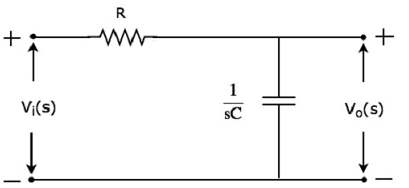It consists of two passive elements resistor and capacitor, which are connected in series. Input voltage is applied across this entire combination and the output is considered as the voltage across capacitor.
Here, Vi(s) and Vo(s) are the Laplace transforms of input voltage, vi(t) and output voltage, vo(t) respectively.
The transfer function of the above network is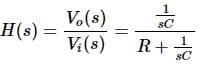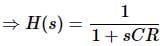Substitute, s = jω in the above equation.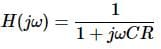Magnitude of transfer function is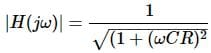• At ω = 0, the magnitude of transfer function is equal to 1.
• At ω = 1/CR , the magnitude of transfer function is equal to 0.707.
• At ω = ∞, the magnitude of transfer function is equal to 0.

Therefore, the magnitude of transfer function of Low pass filter will vary from 1 to 0 as ω varies from 0 to ∞.

High Pass Filter
High pass filter as the name suggests, it allows (passes) only high frequency components. That means, it rejects (blocks) all low frequency components.
The s-domain circuit diagram (network) of High pass filter is shown in the following figure.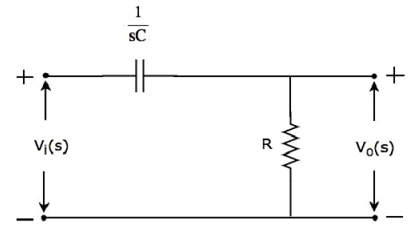It consists of two passive elements capacitor and resistor, which are connected in series. Input voltage is applied across this entire combination and the output is considered as the voltage across resistor.
Here, Vi(s) and Vo(s) are the Laplace transforms of input voltage, vi(t) and output voltage, vo(t) respectively.
The transfer function of the above network is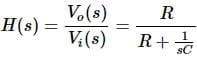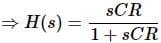Substitute, s = jω in the above equation.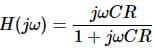Magnitude of transfer function is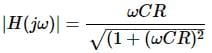• At ω = 0, the magnitude of transfer function is equal to 0.
• At ω = 1/CR, the magnitude of transfer function is equal to 0.707.
• At ω = ∞, the magnitude of transfer function is equal to 1.

Therefore, the magnitude of transfer function of High pass filter will vary from 0 to 1 as ω varies from 0 to ∞.

Band Pass Filter
Band pass filter as the name suggests, it allows (passes) only one band of frequencies. In general, this frequency band lies in between low frequency range and high frequency range. That means, this filter rejects (blocks) both low and high frequency components.
The s-domain circuit diagram (network) of Band pass filter is shown in the following figure.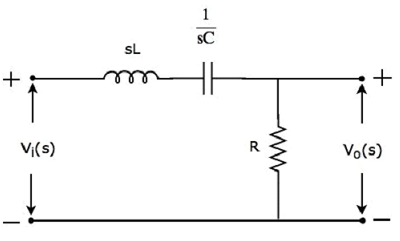It consists of three passive elements inductor, capacitor and resistor, which are connected in series. Input voltage is applied across this entire combination and the output is considered as the voltage across resistor.
Here, Vi(s) and Vo(s) are the Laplace transforms of input voltage, vi(t) and output voltage, vo(t) respectively.
The transfer function of the above network is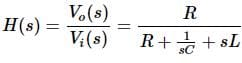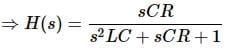Substitute s = jω in the above equation.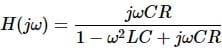Magnitude of transfer function is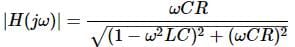• At ω = 0, the magnitude of transfer function is equal to 0.
• At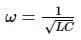, the magnitude of transfer function is equal to 1.
• At ω = ∞, the magnitude of transfer function is equal to 0.

Therefore, the magnitude of transfer function of Band pass filter will vary from 0 to 1 & 1 to 0 as ω varies from 0 to ∞.

Band Stop Filter
Band stop filter as the name suggests, it rejects (blocks) only one band of frequencies. In general, this frequency band lies in between low frequency range and high frequency range. That means, this filter allows (passes) both low and high frequency components.
The s-domain (network) of circuit diagramand stop filter is shown in the following figure.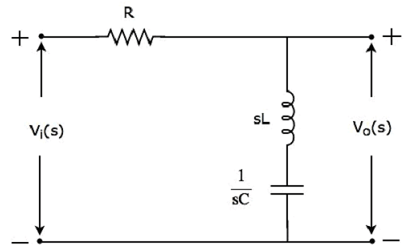It consists of three passive elements resistor, inductor and capacitor, which are connected in series. Input voltage is applied across this entire combination and the output is considered as the voltage across the combination of inductor and capacitor.
Here, Vi(s) and Vo(s) are the Laplace transforms of input voltage, vi(t) and output voltage, vo(t) respectively.
The transfer function of the above network is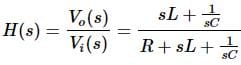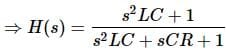Substitute, s = jω in the above equation.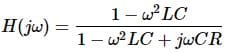Magnitude of transfer function is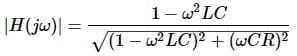• At ω = 0, the magnitude of transfer function is equal to 1.
• At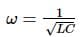the magnitude of transfer function is equal to 0.
• At ω = ∞, the magnitude of transfer function is equal to 1.

Therefore, the magnitude of transfer function of Band stop filter will vary from 1 to 0 & 0 to 1 as ω varies from 0 to ∞.

The document Filters: Network Theory | Network Theory (Electric Circuits) - Electrical Engineering (EE) is a part of the Electrical Engineering (EE) Course Network Theory (Electric Circuits).
All you need of Electrical Engineering (EE) at this link: Electrical Engineering (EE)

## Network Theory (Electric Circuits)

23 videos|63 docs|60 tests

## Network Theory (Electric Circuits)

23 videos|63 docs|60 tests

Track your progress, build streaks, highlight & save important lessons and more!

,

,

,

,

,

,

,

,

,

,

,

,

,

,

,

,

,

,

,

,

,

;